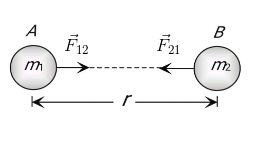# Explain briefly about Newton's law of gravitation

Newton's law of Gravitation-

• According to Newton's law of gravitation, the gravitational force is directly proportional to the product of their masses and inversely proportional to the square of the distance between their centers.

Due to gravitational force, Each particle in this universe attracts every other particle.

The direction of this force is along the line joining the particles.

Let two particles of massesandseparated by a distance r exert  a Force F on each other

And Magnitude of F is given asOr,WhereForceGravitational constantMassesDistance between masses

•  The vector form of formulaAccording to Newton's law of gravitationOrWherePosition vector

Here negative sign indicates that the direction ofis opposite to that ofAndSo,Means Gravitational force between two bodies form an action and reaction pair.

i.e. the forces are equal in magnitude but opposite in direction.

This is in accordance with Newton's third law of motion

### Preparation Products

##### JEE Main Rank Booster 2021

This course will help student to be better prepared and study in the right direction for JEE Main..

₹ 13999/- ₹ 9999/-
##### Rank Booster NEET 2021

This course will help student to be better prepared and study in the right direction for NEET..

₹ 13999/- ₹ 9999/-
##### Knockout JEE Main April 2021 (Easy Installments)

An exhaustive E-learning program for the complete preparation of JEE Main..

₹ 4999/-
##### Knockout NEET May 2021

An exhaustive E-learning program for the complete preparation of NEET..

₹ 22999/- ₹ 14999/-